Chemistry: Atoms First 2e

# Chapter 10

1.

Liquids and solids are similar in that they are matter composed of atoms, ions, or molecules. They are incompressible and have similar densities that are both much larger than those of gases. They are different in that liquids have no fixed shape, and solids are rigid.

3.

They are similar in that the atoms or molecules are free to move from one position to another. They differ in that the particles of a liquid are confined to the shape of the vessel in which they are placed. In contrast, a gas will expand without limit to fill the space into which it is placed.

5.

All atoms and molecules will condense into a liquid or solid in which the attractive forces exceed the kinetic energy of the molecules, at sufficiently low temperature.

7.

(a) Dispersion forces occur as an atom develops a temporary dipole moment when its electrons are distributed asymmetrically about the nucleus. This structure is more prevalent in large atoms such as argon or radon. A second atom can then be distorted by the appearance of the dipole in the first atom. The electrons of the second atom are attracted toward the positive end of the first atom, which sets up a dipole in the second atom. The net result is rapidly fluctuating, temporary dipoles that attract one another (e.g., Ar). (b) A dipole-dipole attraction is a force that results from an electrostatic attraction of the positive end of one polar molecule for the negative end of another polar molecule (e.g., ICI molecules attract one another by dipole-dipole interaction). (c) Hydrogen bonds form whenever a hydrogen atom is bonded to one of the more electronegative atoms, such as a fluorine, oxygen, or nitrogen atom. The electrostatic attraction between the partially positive hydrogen atom in one molecule and the partially negative atom in another molecule gives rise to a strong dipole-dipole interaction called a hydrogen bond (e.g., $HF⋯HF).HF⋯HF).$

9.

The London forces typically increase as the number of electrons increase.

11.

(a) SiH4 < HCl < H2O; (b) F2 < Cl2 < Br2; (c) CH4 < C2H6 < C3H8; (d) N2 < O2 < NO

13.

Only rather small dipole-dipole interactions from C-H bonds are available to hold n-butane in the liquid state. Chloroethane, however, has rather large dipole interactions because of the Cl-C bond; the interaction, therefore, is stronger, leading to a higher boiling point.

15.

−85 °C. Water has stronger hydrogen bonds, so it melts at a higher temperature.

17.

The hydrogen bond between two hydrogen fluoride molecules is stronger than that between two water molecules because the electronegativity of F is greater than that of O. Consequently, the partial negative charge on F is greater than that on O. The hydrogen bond between the partially positive H and the larger partially negative F will be stronger than that formed between H and O.

19.

H-bonding is the principle IMF holding the protein strands together. The H-bonding is between the $N−HN−H$ and $C=O.C=O.$

21.

(a) hydrogen bonding, dipole-dipole attraction, and dispersion forces; (b) dispersion forces; (c) dipole-dipole attraction and dispersion forces

23.

The water molecules have strong intermolecular forces of hydrogen bonding. The water molecules are thus attracted strongly to one another and exhibit a relatively large surface tension, forming a type of “skin” at its surface. This skin can support a bug or paper clip if gently placed on the water.

25.

Temperature has an effect on intermolecular forces: The higher the temperature, the greater the kinetic energies of the molecules and the greater the extent to which their intermolecular forces are overcome, and so the more fluid (less viscous) the liquid. The lower the temperature, the less the intermolecular forces are overcome, and so the less viscous the liquid.

27.

(a) As the water reaches higher temperatures, the increased kinetic energies of its molecules are more effective in overcoming hydrogen bonding, and so its surface tension decreases. Surface tension and intermolecular forces are directly related. (b) The same trend in viscosity is seen as in surface tension, and for the same reason.

29.

1.7 $××$ 10−4 m

31.

The heat is absorbed by the ice, providing the energy required to partially overcome intermolecular attractive forces in the solid and causing a phase transition to liquid water. The solution remains at 0 °C until all the ice is melted. Only the amount of water existing as ice changes until the ice disappears. Then the temperature of the water can rise.

33.

We can see the amount of liquid in an open container decrease and we can smell the vapor of some liquids.

35.

The vapor pressure of a liquid decreases as the strength of its intermolecular forces increases.

37.

As the temperature increases, the average kinetic energy of the molecules of gasoline increases and so a greater fraction of molecules have sufficient energy to escape from the liquid than at lower temperatures.

39.

They are equal when the pressure of gas above the liquid is exactly 1 atm.

41.

approximately 95 °C

43.

(a) At 5000 feet, the atmospheric pressure is lower than at sea level, and water will therefore boil at a lower temperature. This lower temperature will cause the physical and chemical changes involved in cooking the egg to proceed more slowly, and a longer time is required to fully cook the egg. (b) As long as the air surrounding the body contains less water vapor than the maximum that air can hold at that temperature, perspiration will evaporate, thereby cooling the body by removing the heat of vaporization required to vaporize the water.

45.

Dispersion forces increase with molecular mass or size. As the number of atoms composing the molecules in this homologous series increases, so does the extent of intermolecular attraction via dispersion forces and, consequently, the energy required to overcome these forces and vaporize the liquids.

47.

The boiling point of CS2 is higher than that of CO2 partially because of the higher molecular weight of CS2; consequently, the attractive forces are stronger in CS2. It would be expected, therefore, that the heat of vaporization would be greater than that of 9.8 kJ/mol for CO2. A value of 28 kJ/mol would seem reasonable. A value of −8.4 kJ/mol would indicate a release of energy upon vaporization, which is clearly implausible.

49.

The thermal energy (heat) needed to evaporate the liquid is removed from the skin.

51.

1125 kJ

53.

(a) 13.0 kJ; (b) It is likely that the heat of vaporization will have a larger magnitude since in the case of vaporization the intermolecular interactions have to be completely overcome, while melting weakens or destroys only some of them.

55.

At low pressures and 0.005 °C, the water is a gas. As the pressure increases to 4.6 torr, the water becomes a solid; as the pressure increases still more, it becomes a liquid. At 40 °C, water at low pressure is a vapor; at pressures higher than about 75 torr, it converts into a liquid. At −40 °C, water goes from a gas to a solid as the pressure increases above very low values.

57.

(a) gas; (b) gas; (c) gas; (d) gas; (e) solid; (f) gas

59.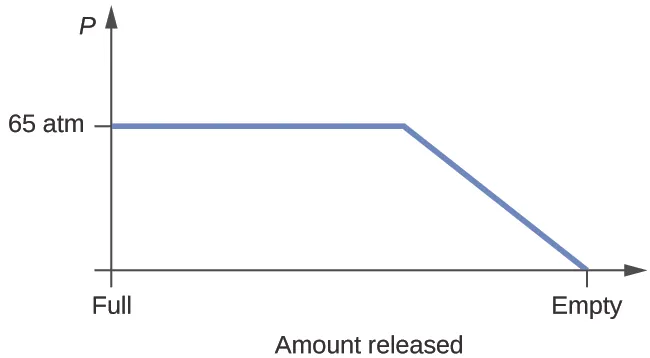61.

Yes, ice will sublime, although it may take it several days. Ice has a small vapor pressure, and some ice molecules form gas and escape from the ice crystals. As time passes, more and more solid converts to gas until eventually the clothes are dry.

63.

(a)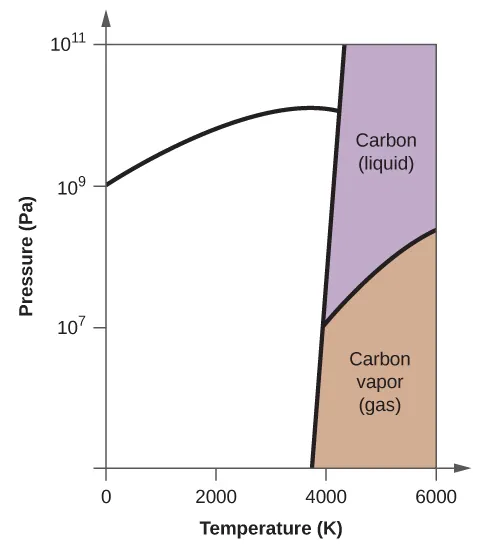(b)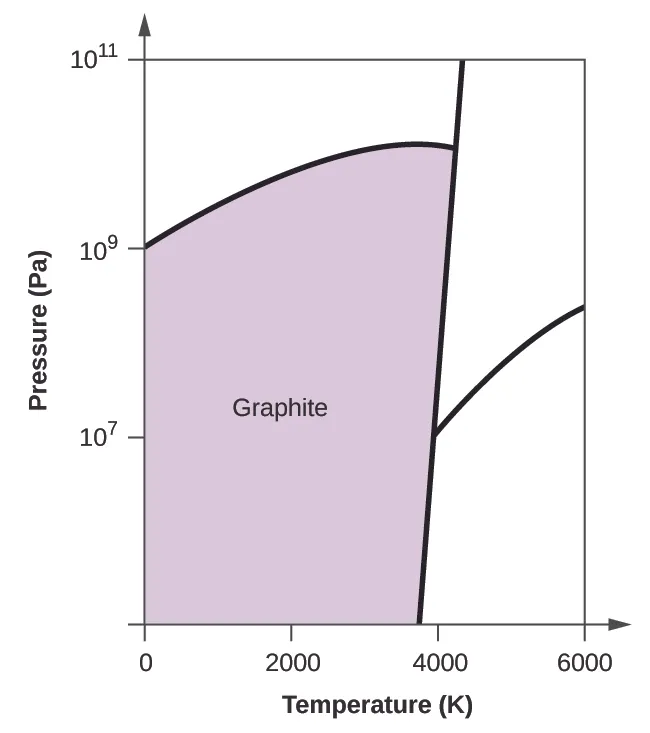(c)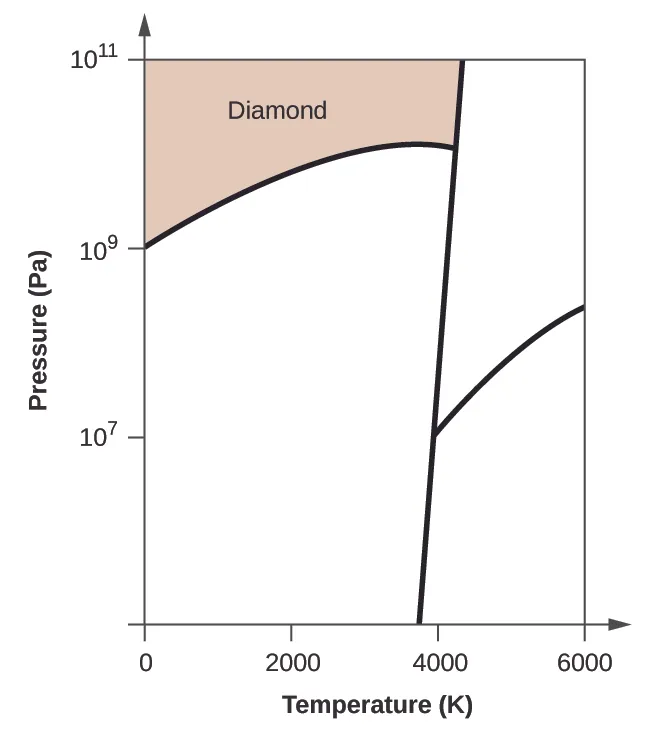(d)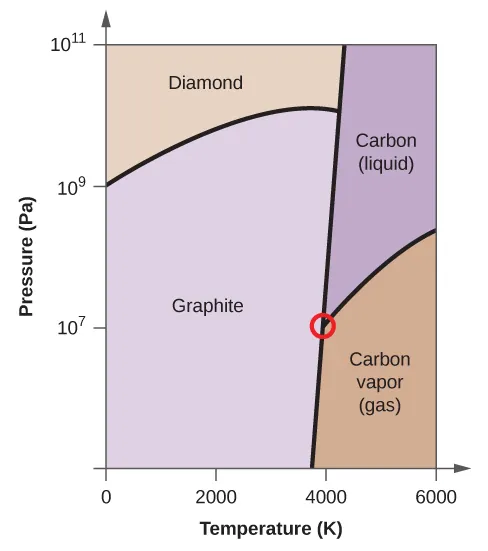(e) liquid phase (f) sublimation

65.

(e) molecular crystals

67.

Ice has a crystalline structure stabilized by hydrogen bonding. These intermolecular forces are of comparable strength and thus require the same amount of energy to overcome. As a result, ice melts at a single temperature and not over a range of temperatures. The various, very large molecules that compose butter experience varied van der Waals attractions of various strengths that are overcome at various temperatures, and so the melting process occurs over a wide temperature range.

69.

(a) ionic; (b) covalent network; (c) molecular; (d) metallic; (e) covalent network; (f) molecular; (g) molecular; (h) ionic; (i) ionic

71.

X = ionic; Y = metallic; Z = covalent network

73.

(b) metallic solid

75.

The structure of this low-temperature form of iron (below 910 °C) is body-centered cubic. There is one-eighth atom at each of the eight corners of the cube and one atom in the center of the cube.

77.

eight

79.

12

81.

(a) 1.370 Å; (b) 19.26 g/cm

83.

(a) 2.176 Å; (b) 3.595 g/cm3

85.

The crystal structure of Si shows that it is less tightly packed (coordination number 4) in the solid than Al (coordination number 12).

87.

In a closest-packed array, two tetrahedral holes exist for each anion. If only half the tetrahedral holes are occupied, the numbers of anions and cations are equal. The formula for cadmium sulfide is CdS.

89.

Co3O4

91.

In a simple cubic array, only one cubic hole can be occupied be a cation for each anion in the array. The ratio of thallium to iodide must be 1:1; therefore, the formula for thallium is TlI.

93.

59.95%; The oxidation number of titanium is +4.

95.

Both ions are close in size: Mg, 0.65; Li, 0.60. This similarity allows the two to interchange rather easily. The difference in charge is generally compensated by the switch of Si4+ for Al3+.

97.

Mn2O3

99.

1.48 Å

101.

2.874 Å

103.

20.2°

105.

1.74 $××$ 104 eV

Order a print copy

As an Amazon Associate we earn from qualifying purchases.